# 555_Spring12_topic22.ppt

30. May 2023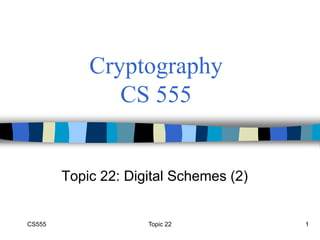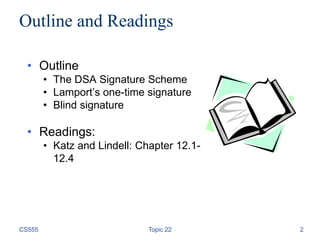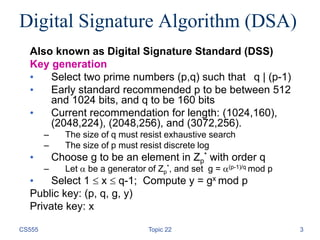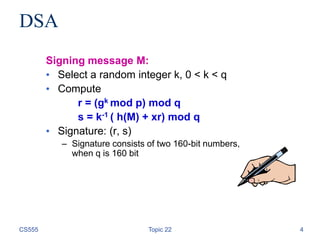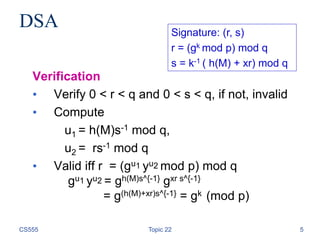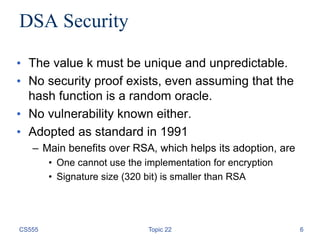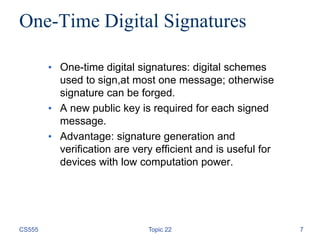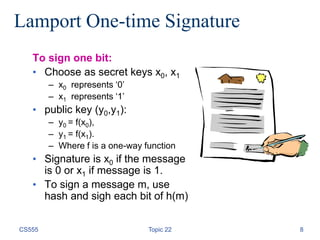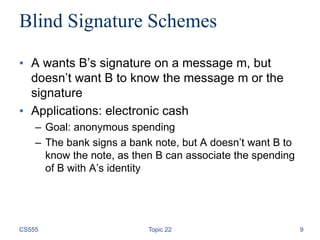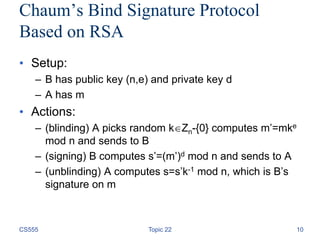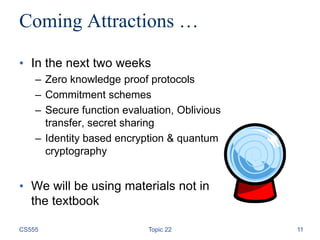1 von 11

### 555_Spring12_topic22.ppt

• 1. CS555 Topic 22 1 Cryptography CS 555 Topic 22: Digital Schemes (2)
• 2. CS555 Topic 22 2 Outline and Readings • Outline • The DSA Signature Scheme • Lamport’s one-time signature • Blind signature • Readings: • Katz and Lindell: Chapter 12.1- 12.4
• 3. Topic 22 3 Digital Signature Algorithm (DSA) Also known as Digital Signature Standard (DSS) Key generation • Select two prime numbers (p,q) such that q | (p-1) • Early standard recommended p to be between 512 and 1024 bits, and q to be 160 bits • Current recommendation for length: (1024,160), (2048,224), (2048,256), and (3072,256). – The size of q must resist exhaustive search – The size of p must resist discrete log • Choose g to be an element in Zp * with order q – Let  be a generator of Zp *, and set g = (p-1)/q mod p • Select 1  x  q-1; Compute y = gx mod p Public key: (p, q, g, y) Private key: x CS555
• 4. Topic 22 4 DSA Signing message M: • Select a random integer k, 0 < k < q • Compute r = (gk mod p) mod q s = k-1 ( h(M) + xr) mod q • Signature: (r, s) – Signature consists of two 160-bit numbers, when q is 160 bit CS555
• 5. Topic 22 5 DSA Verification • Verify 0 < r < q and 0 < s < q, if not, invalid • Compute u1 = h(M)s-1 mod q, u2 = rs-1 mod q • Valid iff r = (gu1 yu2 mod p) mod q gu1 yu2 = gh(M)s^{-1} gxr s^{-1} = g(h(M)+xr)s^{-1} = gk (mod p) Signature: (r, s) r = (gk mod p) mod q s = k-1 ( h(M) + xr) mod q CS555
• 6. DSA Security • The value k must be unique and unpredictable. • No security proof exists, even assuming that the hash function is a random oracle. • No vulnerability known either. • Adopted as standard in 1991 – Main benefits over RSA, which helps its adoption, are • One cannot use the implementation for encryption • Signature size (320 bit) is smaller than RSA CS555 Topic 22 6
• 7. Topic 22 7 One-Time Digital Signatures • One-time digital signatures: digital schemes used to sign,at most one message; otherwise signature can be forged. • A new public key is required for each signed message. • Advantage: signature generation and verification are very efficient and is useful for devices with low computation power. CS555
• 8. Topic 22 8 Lamport One-time Signature To sign one bit: • Choose as secret keys x0, x1 – x0 represents ‘0’ – x1 represents ‘1’ • public key (y0,y1): – y0 = f(x0), – y1 = f(x1). – Where f is a one-way function • Signature is x0 if the message is 0 or x1 if message is 1. • To sign a message m, use hash and sigh each bit of h(m) CS555
• 9. Topic 22 9 Blind Signature Schemes • A wants B’s signature on a message m, but doesn’t want B to know the message m or the signature • Applications: electronic cash – Goal: anonymous spending – The bank signs a bank note, but A doesn’t want B to know the note, as then B can associate the spending of B with A’s identity CS555
• 10. Topic 22 10 Chaum’s Bind Signature Protocol Based on RSA • Setup: – B has public key (n,e) and private key d – A has m • Actions: – (blinding) A picks random kZn-{0} computes m’=mke mod n and sends to B – (signing) B computes s’=(m’)d mod n and sends to A – (unblinding) A computes s=s’k-1 mod n, which is B’s signature on m CS555
• 11. CS555 Topic 22 11 Coming Attractions … • In the next two weeks – Zero knowledge proof protocols – Commitment schemes – Secure function evaluation, Oblivious transfer, secret sharing – Identity based encryption & quantum cryptography • We will be using materials not in the textbook